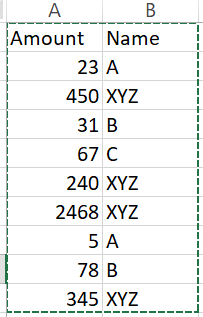# Update a column equally based on amount

Hi,

I have to update name based on the amount column.

For example : If Amount is less than 100, I should split the rows between A, B and C equally. For amount greater than 100 give name as XYZ. PFB picture for reference. Thanks in advanceUse else if activity in for each

if CurrentRow(“Amount”)>100 then assign CurrentRow(“Name”)=“xyz”
if CurrentRow(“Amount”)<33 then assign CurrentRow(“Name”)=“A”
if CurrentRow(“Amount”)>33 and CurrentRow(“Amount”)<66 then assign CurrentRow(“Name”)=“B”

Hi @aishwarya1,

Kindly check this workflow

InputOutput

Workflow

Code

If(CInt(Row(“Amount”).ToString)>100,“XYZ”,
If(CInt(Row(“Amount”).ToString)>50 And CInt(Row(“Amount”).ToString)<100,“C”,
If(CInt(Row(“Amount”).ToString)>25 And CInt(Row(“Amount”).ToString)<50,“B”,
If(CInt(Row(“Amount”).ToString)>0 And CInt(Row(“Amount”).ToString)<25,“A”,
“”))))

Xaml
Sequence.xaml (11.4 KB)

Thanks,
Rajkumar

Hello @aishwarya1 ,
you can try the below:

``````Dim counter as Integer
counter = 0
for each row as Datarow in dt_Input.Rows
if CInt(row("Amount")) > 100 then
row("Name") = "XYZ"
else
if Counter = 0 then
row("Name") = "A"
elseif Counter = 1 then
row("Name") = "B"
elseif Counter = 2 then
row("Name") = "C"
counter = -1
end if
end if
counter = Counter+1
next row
``````

equivalent in UiPath is attached
Test.xaml (10.9 KB)

I tried the approach given by you but getting an output like this.